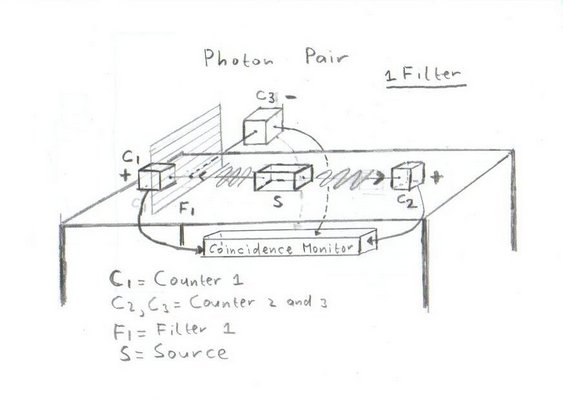## Experiment 2 with Entangled photonsThe assumption is:
• That the left photon moves towards the left and is polarised in the horizontal plane .
• That the right photon moves towards the right and is also polarised in the horizontal plane .
The idea behind this experiment is to test the sequence of events when you insert a two channel polariser in the left beam and you leave the right beam undisturbed with the aid of a Coincidence Monitor.

The result of the test will be 2 sequence of events, showing the arrival times of the photons.

```Counter 1: x    x x    x       x     x        x    x  x       tn1 =  9
Counter 3:   x       x   x  x    x      x   x      x    x   x tn3 = 10
Counter 2: x x  x    x x    x  x x   x  x x x x  x x  x x   x tn2 = 18
```
The above pattern shows no difference for the right beam (counter 2)
In total 20 photon pairs are created and 2 are missing.
For the left beam there is a clear difference.
• Approximately 50% goes to counter in the + direction and almost 50% goes to counter 3 in the - direction.
• At 2 occasions nothing is counted and at one occasion a photon is counted at both counters.